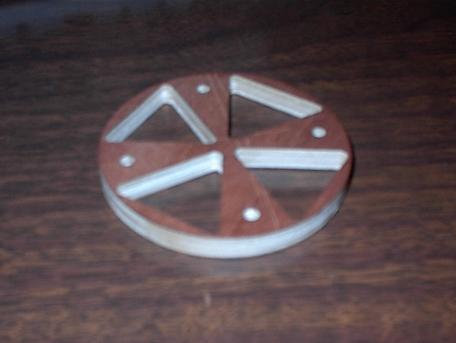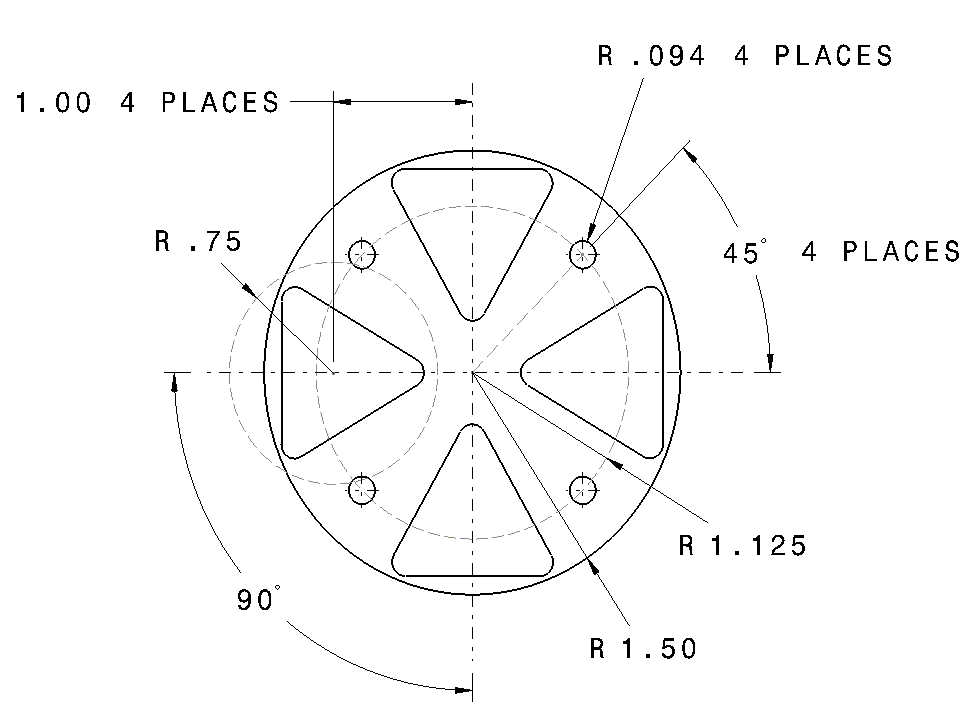Simple CNC Project_1How to Step by Step:

- 6 Steps in all

1. Bolt Hole Pattern
• Tool Dia = .1875
• Number of Holes = 4
• Start Angle = 45
• X Offset From Zero = 0
• Y Offset From Zero
• Z Drill Depth = -.25

2. Polygonshapes - 4 Triangles

 1. Triangle Tool Dia = .1875 Circle Rad = .75 Tool Position = InSide Start Angle = 0 Number of Side = Triangle X Offset From Zero = -1.0 Y Offset From Zero = 0 Z Depth = -.05 3. Triangle Tool Dia = .1875 Circle Rad = .75 Tool Position = InSide Start Angle = 90 Number of Side = Triangle X Offset From Zero = 0 Y Offset From Zero = 1.0 Z Depth = -.05 2. Triangle Tool Dia = .1875 Circle Rad = .75 Tool Position = InSide Start Angle = 180 Number of Side = Triangle X Offset From Zero = 1.0 Y Offset From Zero = 0 Z Depth = -.05 4. Triangle Tool Dia = .1875 Circle Rad = .75 Tool Position = InSide Start Angle = 270 Number of Side = Triangle X Offset From Zero = 0 Y Offset From Zero = -1.0 Z Depth = -.05

3. Circle Profile

• Circle Cutting = OutSide
• Number of Steps in Z = 5
• Tool Dia = .1875
• X Offset From Zero = 0
• Y Offset From Zero = 0
• Z Depth per Step = -.05

Once each of the programs are created your ready to set up your stock and start cutting.

Email me

Simple CNC - CNC Machine - Gcode - Conversational - CNC Programming - CNC Software - Bolt Hole Circle - Pocket - Circle - Profile - Drill

Simple CNC - CNC Machine - Gcode - Conversational - CNC Programming - CNC Software - Bolt Hole Circle - Pocket - Circle - Profile - Drill

Simple CNC - CNC Machine - Gcode - Conversational - CNC Programming - CNC Software - Bolt Hole Circle - Pocket - Circle - Profile - Drill

Simple CNC - CNC Machine - Gcode - Conversational - CNC Programming - CNC Software - Bolt Hole Circle - Pocket - Circle - Profile - Drill

Simple CNC - CNC Machine - Gcode - Conversational - CNC Programming - CNC Software - Bolt Hole Circle - Pocket - Circle - Profile - Drill

Simple CNC - CNC Machine - Gcode - Conversational - CNC Programming - CNC Software - Bolt Hole Circle - Pocket - Circle - Profile - Drill

Simple CNC - CNC Machine - Gcode - Conversational - CNC Programming - CNC Software - Bolt Hole Circle - Pocket - Circle - Profile - Drill

Simple CNC - CNC Machine - Gcode - Conversational - CNC Programming - CNC Software - Bolt Hole Circle - Pocket - Circle - Profile - Drill